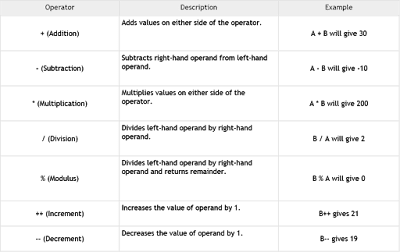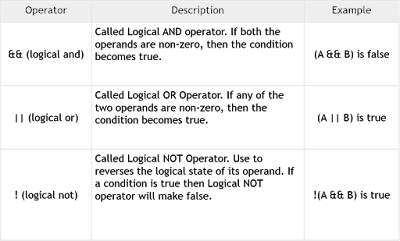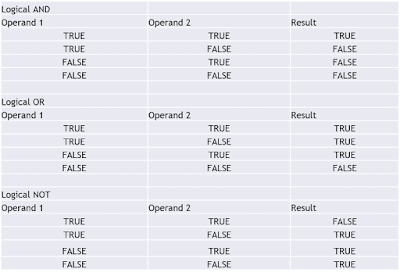## Pages

### Operators in Java

What is an Operator?

Operator in java is a symbol that is used to perform operations. For example: +, -, *, / etc.
Java provides a rich set of operators to manipulate variables.We will discuss about below operators in this post.

1.Arithmetic Operators
2.Relational Operators
3.Logical Operators
4.Assignment Operators
5.Misc Operators

Arithmetic Operators

Java arithmetic operators are used to perform addition, subtraction, multiplication, and division. They are used to perform basic mathematical operations.Arithmetic Operators

Relational Operators

Relational Operators returns Boolean/Logical result.

== (equal to)
!= (not equal to)
> (greater than)
< (less than)
>= (greater than or equal to)
<= (less than or equal to)

Logical Operators:

There are three logical operators given below:Logical OperatorsHow Logical Operator Works

Assignment Operators

Below are the Assignment operators are given with explanation:Assignment Operators with Examples

Misc Operators

Conditional Operator - Conditional operator is also known as the ternary operator. This operator consists of three operands and is used to evaluate Boolean expressions. The goal of the operator is to decide, which value should be assigned to the variable. The operator is written as −

variable x = (expression) ? value if true : value if false

instanceof Operator - This operator is used only for object reference variables. The operator checks whether the object is of a particular type (class type or interface type). instanceof operator is written as −

( Object reference variable ) instanceof  (class/interface type)

To get more details please watch below youtube video and Subscribe the channel.